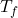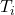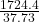## A 9.02-g sample of liquid water at 40.3 °C is heated by the addition of 203.93 J of energy. The final temperature of the water is ________ °

Question

A 9.02-g sample of liquid water at 40.3 °C is heated by the addition of 203.93 J of energy. The final temperature of the water is ________ °C. The specific heat capacity of liquid water is 4.184 J/gK.

in progress 0
2 weeks 2021-08-30T01:19:51+00:00 1 Answers 0 views 0

45.70 C

Explanation:

We’ll use the formula for specific heat Q=mcΔT. Let’s first check out units and look at the values we have.

Q=heat= 203.93 J

m=mass= 9.02g

c= specific heat= 4.184 J/g K

ΔT= Change in temperature= Final temp. – initial temp. =Let’s plug in what we know.

203.93 J = (9.02g)(4.184)(– 40.3 C)

We’ll just solve this algebraically to solve for. It’s like solving for x, just a different variable. It’s easier to do the more complicated side first and then start moving your numbers to the other side.

203.93 = (37.73)(– 40.3) We’ll multiply 37.73 byAND 40.3 so we’ll get…

203.93 = (37.73Tf – 1520.5)

Let’s start moving numbers to the other side

203.93 + 1520.5 = 37.73Tf

1724.4 = 37.73Tf=45.70 C=This makes sense with our problem since they heated water, so we should see an increase in temperature.